# Engineering Mechanics - KOP: Impulse and Momentum

### Exercise :: KOP: Impulse and Momentum - General Questions

• KOP: Impulse and Momentum - General Questions
16.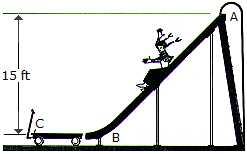A girl having a weight of 40 lb slides down the smooth slide onto the surface of a 20-lb wagon. Determine the speed of the wagon at the instant the girl stops sliding on it. If someone ties the wagon to the slide at B, determine the horizontal impulse the girl will exert at C in order to stop her motion. Neglect friction and assume that the girl starts from rest at the top of the slide, A.

 A. vwagon = 20.7 ft/s Imp = 38.6 lb-s B. vwagon = 31.1 ft/s Imp = 38.6 lb-s C. vwagon = 31.1 ft/s Imp = 57.9 lb-s D. vwagon = 20.7 ft/s Imp = 57.9 lb-s

Explanation:

No answer description available for this question. Let us discuss.

17.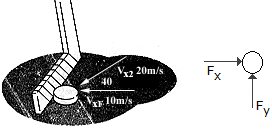A hockey puck is traveling to the left with a velocity of v1 = 10 m/s when it is struck by a hockey stick and given a velocity of v2 = 20 m/s as shown. Determine the magnitude of the net impulse exerted by the hockey stick on the puck. The puck has a mass of 0.2 kg.

 A. Imp = 6.00 N-s B. Imp = 2.00 N-s C. Imp = 2.78 N-s D. Imp = 5.68 N-s

Explanation:

No answer description available for this question. Let us discuss.

18.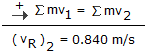A rifle has a mass of 2.5 kg. If it is loosely gripped and a 1.5-g bullet is fired from it with a muzzle velocity of 1400 m/s, determine the recoil velocity of the rifle just after firing.

 A. vrifle = 0.840 m/s B. vrifle = 0.525 m/s C. vrifle = 2.33 m/s D. vrifle = 1.400 m/s

Explanation:

No answer description available for this question. Let us discuss.

19.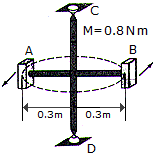The two blocks A and B each have a mass of 500 g. The blocks are fixed to the horizontal rods and their initial velocity is 2 m/s in the direction shown. If a couple moment of M = 0.8 N • m is applied about CD of the frame, determine the speed of the block in 4 s. The mass of the supporting frame is negligible and its free to rotate about CD.

 A. v2 = 10.67 m/s B. v2 = 23.3 m/s C. v2 = 22.3 m/s D. v2 = 12.67 m/s

Explanation:

No answer description available for this question. Let us discuss.

20.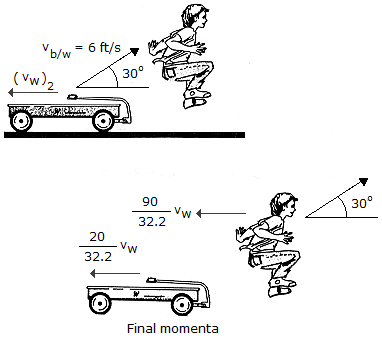A boy, having a weight of 90 lb, jumps off a wagon with a relative velocity of vb/w = 6 ft/s. If the angle of jump is 30°, determine the horizontal velocity (vw)2 of the wagon just after the jump. Originally both the wagon and the boy are at rest. Also, compute the total average impulsive force that all four wheels of the wagon exert on the ground of the boy jumps off in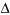t = 0.8s. The wagon has a weight of 20 lb.

 A. vwagon = 23.4 ft/s Nwheel = 110.0 lb B. vwagon = 23.4 ft/s Nwheel = 120.5 lb C. vwagon = 4.25 ft/s Nwheel = 120.5 lb D. vwagon = 4.25 ft/s Nwheel = 110.0 lb